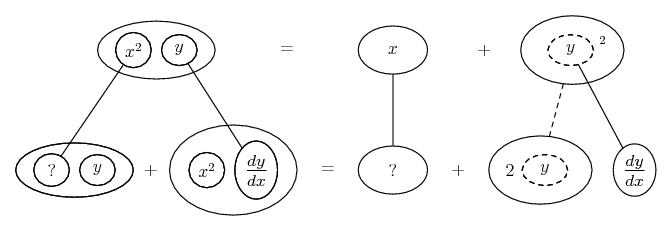# Thread: Implicit differenriarion

1. ## Implicit differenriarion

Hello All,

I'm working on differentiations right now and I'm having a little trouble trying to fully understand. I did this one, but I'm not sure if it's right.

$\displaystyle x^2y=x+y^2$

$\displaystyle \frac{d}{dx}[x^2y]=\frac{d}{dx}[x]+\frac{d}{dx}[y^2]$

$\displaystyle 2xy\frac{dy}{dx}[y]=x+\frac{dy}{dx}[2y]$

$\displaystyle \frac{dy}{dx}(y-2y)=x-2xy$

$\displaystyle \frac{dy}{dx}=\frac{x-2xy}{y-2y}$

Did I do it right?

2.Originally Posted by drkidd22Hello All,

I'm working on differentiations right now and I'm having a little trouble trying to fully understand. I did this one, but I'm not sure if it's right.

$\displaystyle x^2y=x+y^2$

$\displaystyle \frac{d}{dx}[x^2y]=\frac{d}{dx}[x]+\frac{d}{dx}[y^2]$ Good

$\displaystyle 2xy\frac{dy}{dx}[y]=x+\frac{dy}{dx}[2y]$ Product rule on left hand side... and, what is the derivative of x with respect to x?

$\displaystyle \frac{dy}{dx}(y-2y)=x-2xy$

$\displaystyle \frac{dy}{dx}=\frac{x-2xy}{y-2y}$

Did I do it right?

Sorry I can't get the red to work, will post more in a tic

Edit:

Just in case a picture helps...... where... is the product rule, andthe chain rule. (Straight continuous lines differentiate downwards with respect to x, the straight dashed line similarly but with respect to the dashed balloon expression which is the inner function for the chain rule.)

_________________________________
Don't integrate - balloontegrate!

http://www.ballooncalculus.org/forum/top.php

Draw balloons with Latex: http://www.ballooncalculus.org/asy/doc.html

3. The LHS is incorrect--use product rule:

d(x^2y)/dx = 2xy + x^2dy/dx

The Rhs is 1 +2ydy/dx

2xy + x^2dy/dx = 1 +2ydy/dx

now finish

4. In general for implicit differentiation

1. differentiate fns of x normally d(x^2)/dx = 2x

2. when differentiating fns of y diff with respect to y and multiply by dy/x

d(y^2)/dx = 2y dy/dx

5. $\displaystyle \frac{dy}{dx}=\frac{1-2xy}{x^2-2y}$

6. Yep

7. thanks, I liked the diagram you posted. Look my other thread coming up. I got another one.

#### Search Tags

differenriarion, implicit